# MLF - Machine Learning Typologies

Last updated: April 9th, 2020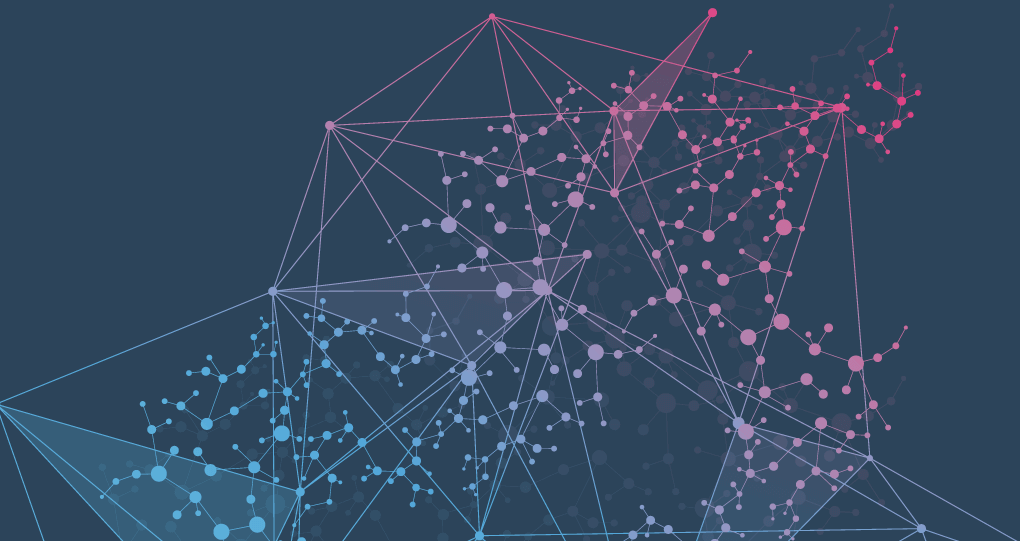# Machine Learning typologies¶

In a world saturated by artificial intelligence, machine learning, and over-zealous talk about both, it is interesting understand and identify the types of machine learning we may encounter.

There some variations of how to define the types of Machine Learning and its algorithms, in this lesson we'll analyze the most used typologies we can find.## Depending on the content of the data¶

Depending on the content of the dataset we are going to use we can divide Machine learning types in Supervised learning, Unsupervised learning, Semi-supervised learning and Reinforcement learning.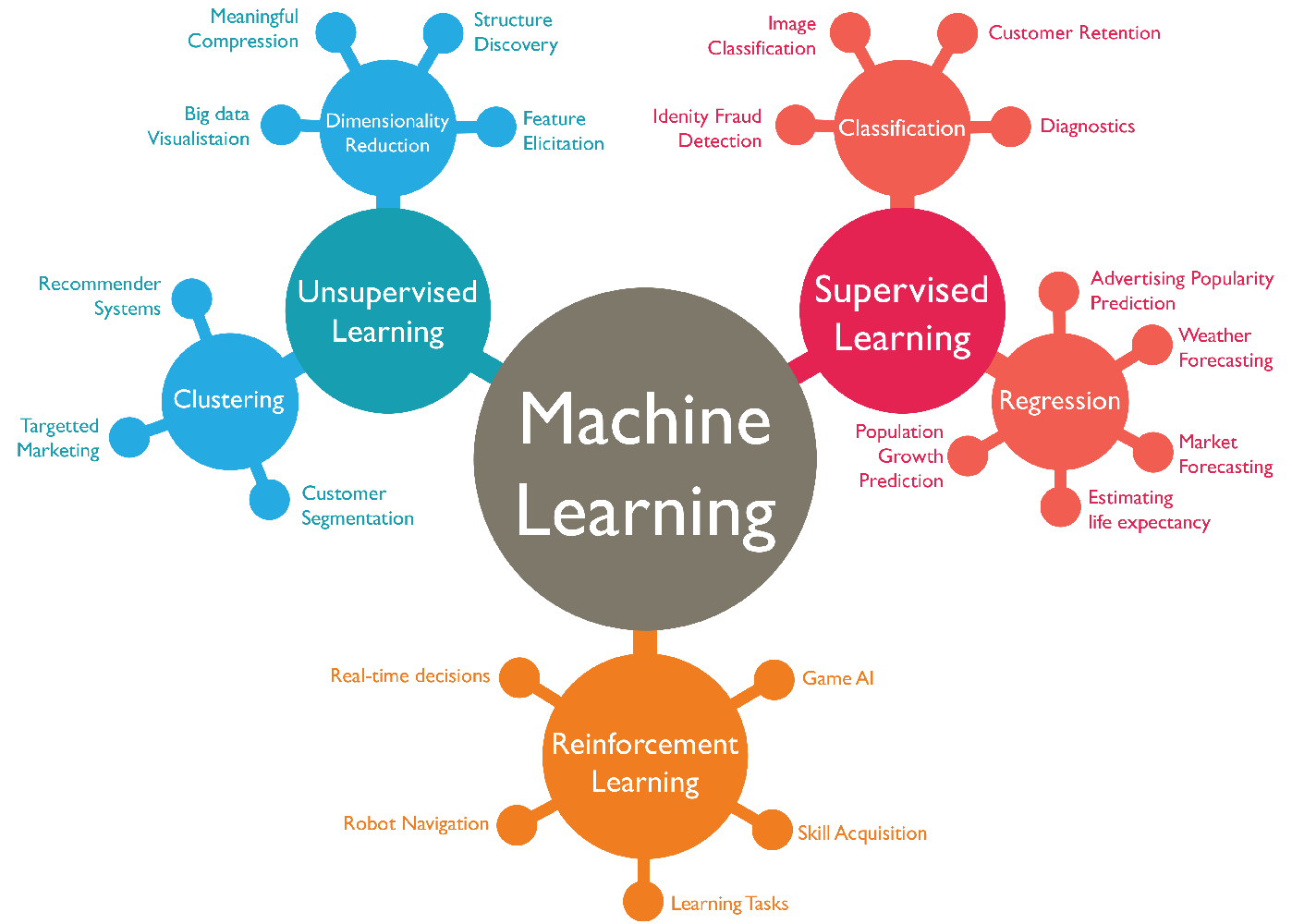### Supervised learning¶

Supervised learning is the most popular paradigm for machine learning, which has been studied the most and for which there is the largest number of available algorithms already developed. It is the easiest to understand and the simplest to implement.

Supervised learning algorithms try to model relationships and dependencies between the target prediction output ($Y$) and the input features ($x$) such that we can predict the output values for new data based on those relationships which it learned from the previous datasets. It is often described as task-oriented because of this.

$$Y = f(x)$$$$type = f([color, weight])$$

The goal is to approximate the mapping function so well that when you have new input data ($x$) that you can predict the output variables ($Y$) for that data.

By having "tagged" data it's easy to evaluate the performance of any algorithm since the prediction result can be compared with reality, ground truth.

Let's see a quick example:

color weight type
red 98g apple
green 105g apple
yellow 122g banana
red 103g apple
peach 117g orange
red 95g apple
green 101g ???

It is highly focused on a singular task, feeding more and more examples to the algorithm until it can accurately perform on that task.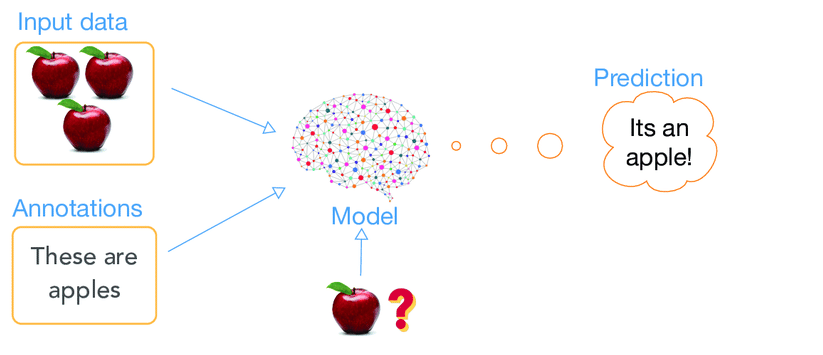### Unsupervised learning¶

Unsupervised learning is where you only have input data ($x$) and no corresponding output variables. All the observations are supplied to the learning algorithm that will assign each of them to a group. Unsupervised learning is very much the opposite of Supervised learning. It features no labels.

In this case, generally, we don't have a ground truth so the performance of these algorithms is usually more complex.

Let's see a quick example:

color weight
red 98g
green 105g
yellow 122g
red 103g
peach 117g
red 95g

In this case we don't have the annotations (target variable) available we want to predict.

Because unsupervised learning is based upon the data and its properties, we can say that unsupervised learning is data-driven. The outcomes from an unsupervised learning task are controlled by the data and the way its formatted.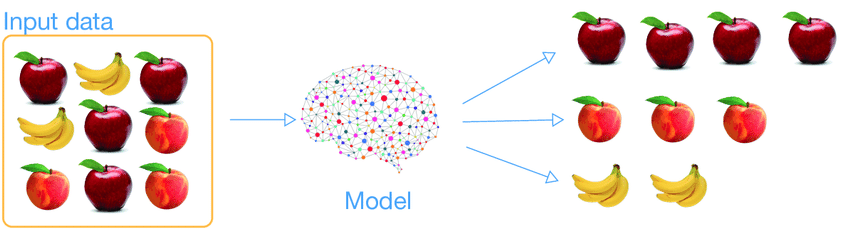### Semi-supervised learning¶

Semi-supervised learning has also been described, and is a hybridization of supervised and unsupervised techniques.

In the previous two types, either there are no labels ($Y$) for all the input data ($x$) in the dataset or labels are present for all the data. Semi-supervised learning falls in between these two.

In many practical situations, the cost to label is quite high, since it requires skilled human experts to do that. So, in the absence of labels in the majority of the observations but present in few, semi-supervised algorithms are the best candidates for the model building. These methods exploit the idea that even though the group memberships of the unlabeled data are unknown, this data carries important information about the group parameters.

### Reinforcement learning¶

Reinforcement learning is fairly different when compared to supervised and unsupervised learning. Where we can easily see the relationship between supervised and unsupervised (the presence or absence of labels), the relationship to reinforcement learning is a bit murkier.

This method aims at using observations gathered from the interaction with the environment to take actions that would maximize the reward or minimize the risk. Reinforcement learning algorithm (called the agent) continuously learns from the environment and its specific context in an iterative way.

Reinforcement learning is very behavior driven. It has influences from the fields of neuroscience and psychology. If you've heard of Pavlov's dog, then you may already be familiar with the idea of reinforcing an agent, albeit a biological one.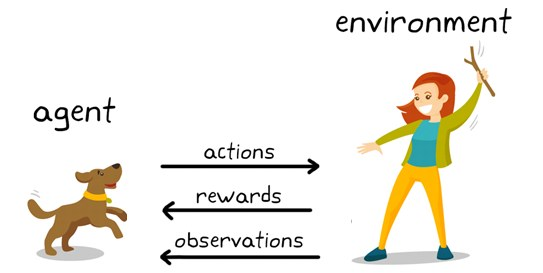Simple reward feedback is required for the agent to learn its behavior; this is known as the reinforcement signal.

The agent will make a lot of mistakes in the beginning, but so long as we provide some sort of signal to the algorithm that associates good behaviors with a positive signal and bad behaviors with a negative one, the agent will be reinforced to prefer good behaviors over bad ones. Over time, our learning algorithm will learn to make less mistakes than it used to, learning from its own mistakes.## Depending on the objective we are looking to model¶

Depending on the objective we are looking to model we can divide Machine learning types in Regression, Classification, Clustering and Association.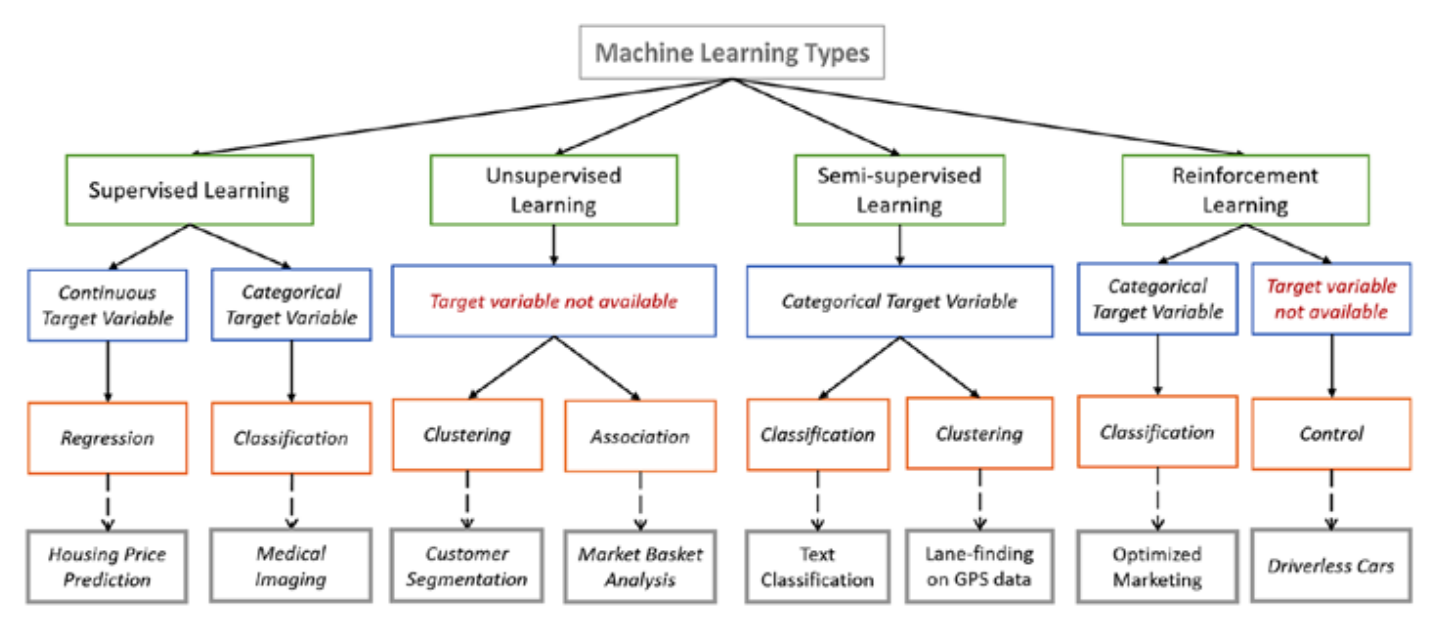### Regression¶

Regression is the task of predicting the value of a given continuous feature based on the values of other features in the data, assuming a linear or nonlinear model of dependency.

A regression algorithm may predict a discrete value, but the discrete value in the form of an integer quantity.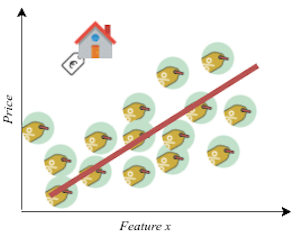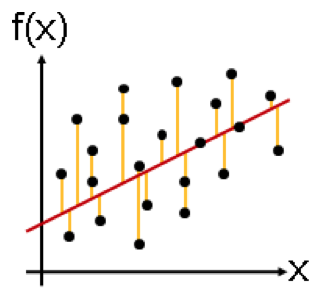Examples of objective variables for regression: house prices, years of life, cholesterol in blood, number of students enrolled, average grade of record, etc.

### Classification¶

Classification is the task of predicting the value of a given discrete class label based on the values of other features in the data.

A classification algorithm may predict a continuous value, but the continuous value is in the form of a probability for a class label.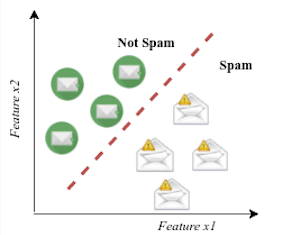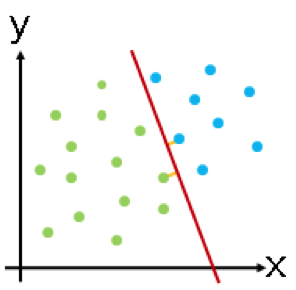Examples of objective variables for classification: 1/0, True/False, abandonment/churn, purchase/no purchase, spam/non-spam, recognition of written numbers, etc.

### Clustering¶

A clustering problem is where you want to discover the inherent groupings in the data, such as grouping customers by purchasing behavior.

Clustering would be fed a lot of data and given the tools to understand the properties of the data. From there, it can learn to group, cluster, and/or organize the data in a way such that a human (or other intelligent algorithm) can come in and make sense of the newly organized data.

The algorithm will seek to obtain groups with maximum internal homogeneity (two observations belonging to the same group should be very similar) and maximum external heterogeneity (two observations belonging to different groups must be very different).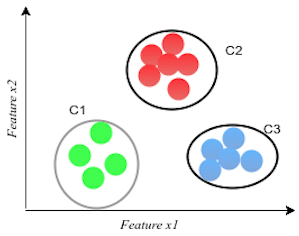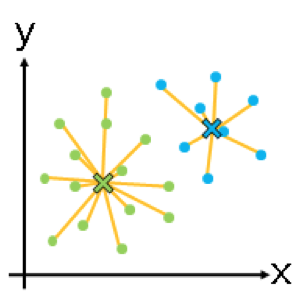### Association¶

Association rules allow you to establish associations amongst data objects inside large databases.

This unsupervised technique is about discovering interesting relationships between variables in large databases. For example, people that buy a new home most likely to buy new furniture.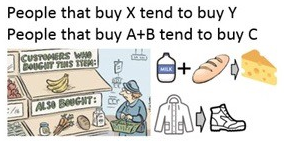## Depending on the periodicity of training¶

Depending on the periodicity of training we can divide Machine learning types in Batch learning and Online learning.

### Batch learning¶

We talk about batch or static machine learning when using the complete available dataset to training our models in a monolithic way.

Once the model is trained, it remains a constant throughout its use which, depending on your ability to generalize, can cause you to lose predictive ability over time.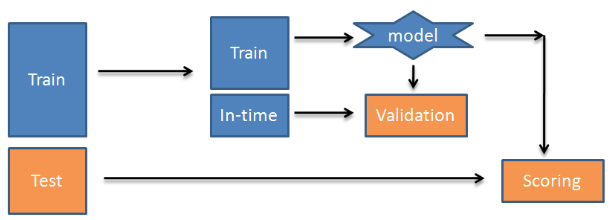It is the least expensive way to build machine learning systems since the training (even if you have more data) is done only once.

It is indicated when you have enough training data and we don't expect abrupt changes in the patterns found in the data.

### Online learning¶

We talk about online or dynamic machine learning when the model keeps training periodically as new observations appear.

The model is retraining "live" so the predictions will always use all observations, old and new ones, that have occurred to date. This will take to more precise models because of considering more recent data.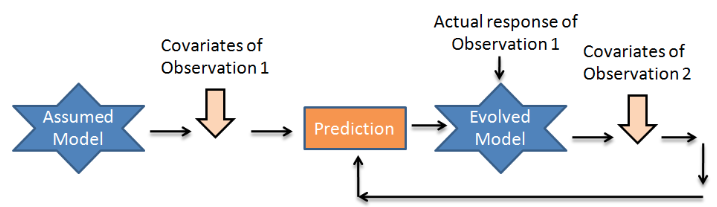However, it is an expensive way to build machine learning systems since that training must be carried out continuously and there is a risk of overfit the models.

It is indicated when you do not have enough training data and/or are expected abrupt changes in the patterns found in the data.